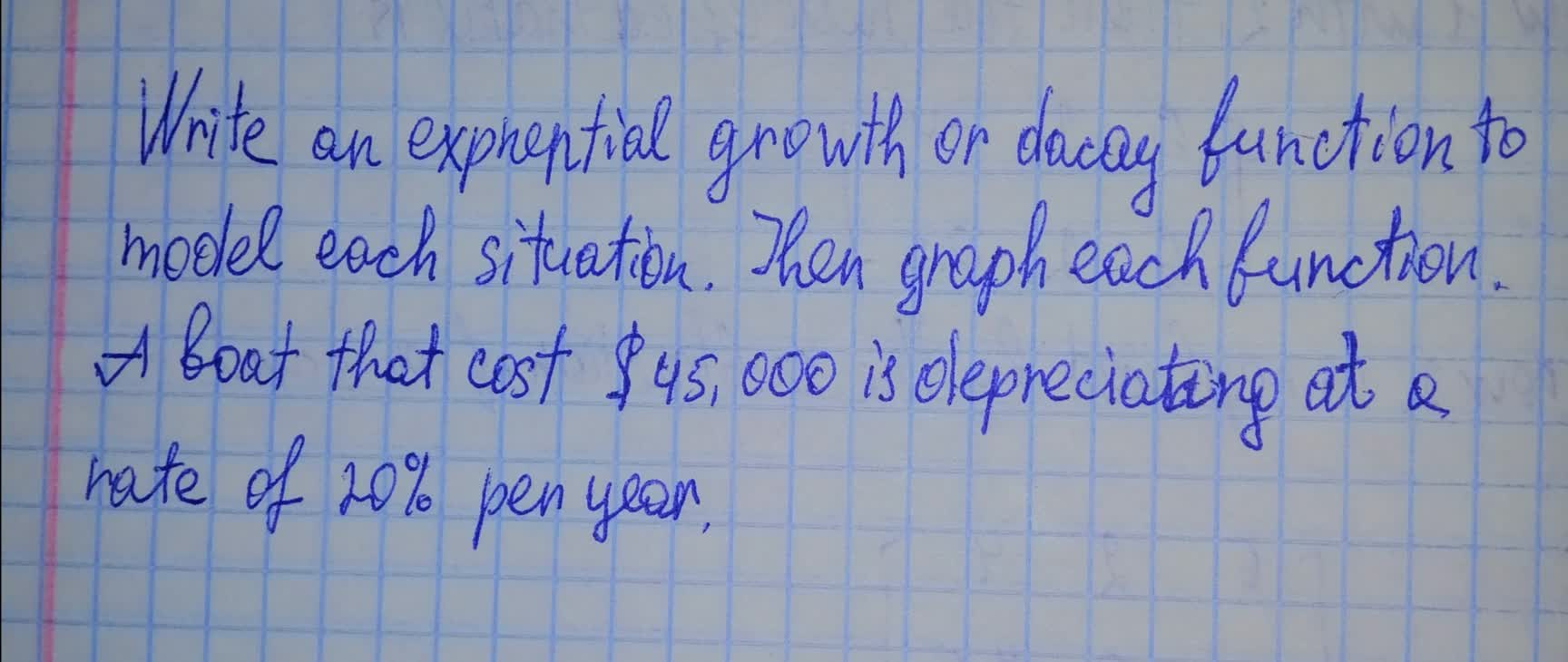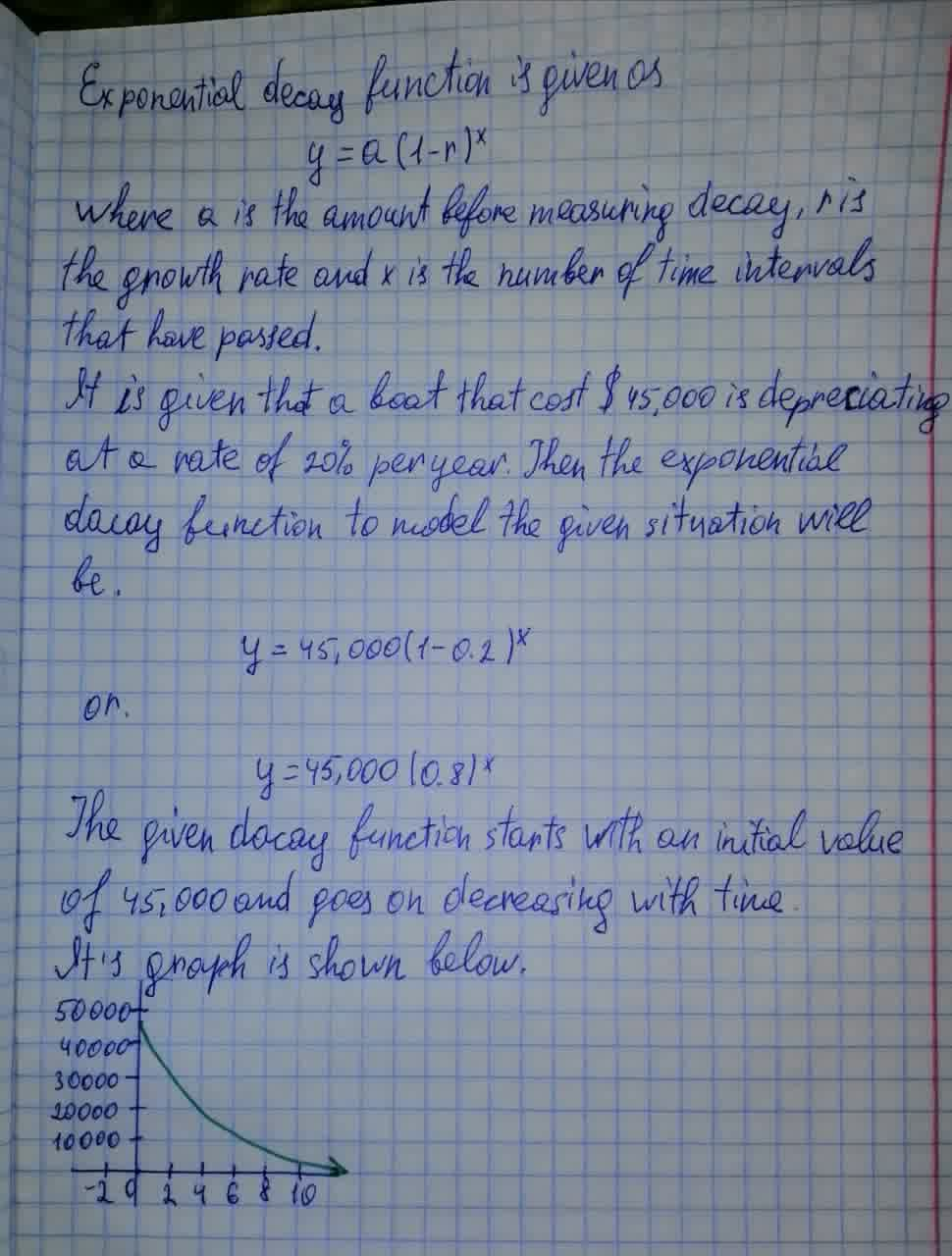# Write an exponential growth or decay function to model each situation. A boat that cost $45,000 is depreciating at a rate of 20% per year.melodykap 2021-07-27 Answered Write an exponential growth or decay function to model each situation. Then graph each function. A boat that cost$45,000 is depreciating at a rate of 20% per year.• Questions are typically answered in as fast as 30 minutes

### Plainmath recommends

• Get a detailed answer even on the hardest topics.
• Ask an expert for a step-by-step guidance to learn to do it yourself.saiyansruleA

Solved this problem in the photo below